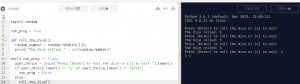# Python : Roll the Dice Multiple Times

We would like to expand the code from the previous exercise to allow multiple rolls of the dice without having to re-run the program each time. This exercise introduces the following concepts:

• Whitespace
• String Manipulation
• Functions
• While Loops
• If Control Statements

```import random

run_prog = True

def roll_the_dice():
random_number = random.randint(1,6)
print("The dice rolled: " + str(random_number))

while run_prog == True:
user_choice = input("Press [Enter] to roll the dice or [x] to exit ").lower()
if user_choice == "x":
run_prog = False
else:
roll_the_dice()
```• Line 3 creates a variable named ‘run_prog’ and we use this later on line 9 to determine if the dice should be rolled again or if the program should exit
• Lines 5-7 we create our own function using the ‘def’ to define the name of the function as ‘roll_the_dice’. This means that each time we use ‘roll_the_dice()’ the lines of code inside our function are run. Notice that any lines indented to the right form part of the function.
• On line 9 we use another special command ‘while’ . This allows us to ‘loop’ indefinitely. Continuously running the code underneath the ‘while’ while a particular condition remains true. For our while loop we will keep running until something changes run_prog from true to false.
• On line 10 we ask the user for some input. We also use string manipulation convert the input to lower case – just in case the user puts a capital X in. Computers view ‘x’ and ‘X’ as two different characters.
• On line 11 we make a decision using an IF statement. We say that ‘if‘ the user supplied an ‘x’ into the ‘user_choice’ variable ‘Then‘ run the code below i.e. set ‘run_prog’ to be false ( this has the effect of stopping the ‘while’ loop created on line 9).
If‘ the user_choice variable is not an ‘x’ then the ‘else‘ code will be run i.e. we run the function defined earlier and named ‘roll_the_dice’.
• Notice that the code inside the if statement is indented to the right to show the lines that belong to the ‘if’ and the lines that belong to the ‘else’

## What Next

• You could expand this game to create games like ‘frustration’ or ‘snakes and ladders’
• Next Challenge: Create a python program that will generate usernames by random combining adjectives and nouns (exercise here)